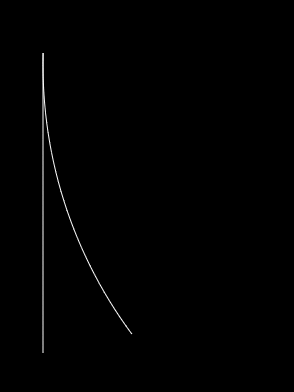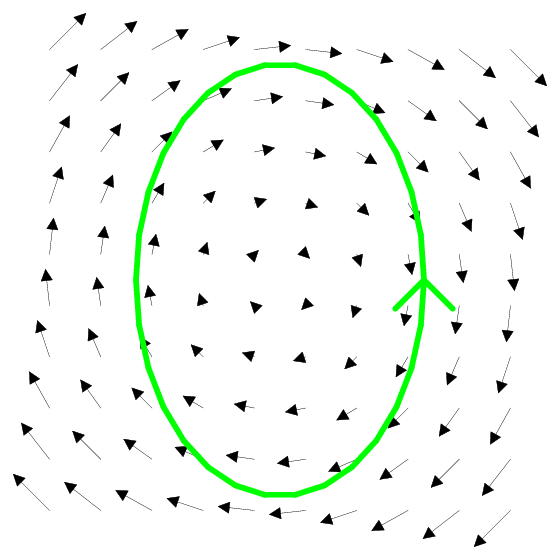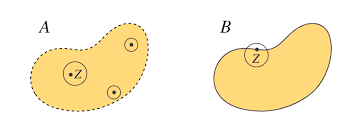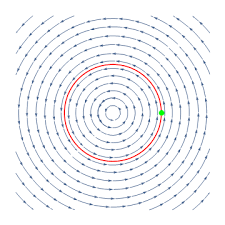## What it’s like to be a PhD student of B. Halstead

Apparently we have some readers in the community of folks with whom Mr. Tetra Halstead associates. Good morning to you all.

Every other school day from 1:30–3:00, the four of us (J. Brewster, B. Halstead, D. Staudinger, D. A-M. Trần) meet in our school’s library or other sufficiently mathematical location and do maths. Previously, we had been going through coursework altogether, under the careful guidance of Mr. Halstead. During that time we worked on introductory Abstract Algebra (Algebra: Chapter 0 by Paolo Aluffi), Real Analysis (Understanding Analysis by Stephen Abbot), and Topology (Topology by James R. Munkres). As of now, we have broken off into working on our own topics which interest us.

I, D. Staudinger, have been occupying lots of Mr. Halstead’s time working on a variety of topics. I have worked a bit on Differential Geometry/Topology and Analysis. Mr. Brewster, being an engineer, has been working more with Linear Algebra and its application with the approximation of bridges and heat transfer and such topics. Mr. Trần has been working with topics in logic and algebra.

Our goal with this independent study is about learning the maths that we are interested in and maybe applicable in our future. I, D. Staudinger, plan to study pure maths in college. Mr. Trần has had a long-standing fascination with logic. Mr. Brewster, being an engineer, is using this time to hone the mathematical basis for simulations and the underpinning of lots of real engineering calculations.

The blog will be updated shortly when we decide where we will all go to college.

## Making Shapes with Quotients

An understanding of quotients is an important prerequisite for creating various surfaces in Euclidean spaces with topology.

As a review, a quotient formalizes the idea of “gluing” points together by any points with the same remainder being equivalent in the quotient.

The torus, which can be thought of as the surface of a doughnut, is a classic example of what can be done with quotients in topology. The solution to the exercise at the of the article on quotients is that $$\mathbb{R}\times \mathbb{R}/\mathbb{Z}\times \mathbb{Z}$$ creates a torus. As covered in the circle example in that same article, the points that are an integer apart are glued together when quotienting by $$\mathbb{Z}$$. This is visualized as starting with a unit square (since all other points can be sent to some point in or on the boundary of that) and then gluing opposite sides together.To physically construct a torus, one glues ends of a rectangle together to create a cylinder and then glues the ends together to create a torus. By doing $$\mathbb{R}\times \mathbb{R}/\mathbb{Z}\times \mathbb{Z}$$, these operations are done simultaneously and a torus is created.

An alternative way to speak of a torus is as $$S^1 \times S^1$$. Two unique circles can be drawn on a torus, as seen below.Since $$S^1 = \mathbb{R}/\mathbb{Z}$$, the reader should check for understanding of products and quotients that $$\mathbb{R}/\mathbb{Z}\times \mathbb{R}/\mathbb{Z} = \mathbb{R}\times \mathbb{R}/\mathbb{Z}\times \mathbb{Z}$$ makes sense.

To get to more interesting shapes than relatively common solids, consider the Möbius strip. Using the physical construction of the Möbius strip as a guide, we can figure out the topological construction. To make a Möbius strip, one takes a rectangle, flips one side, and connects it to the opposite side. This creates a 3D shape with one side and one boundary curve. An interesting (albeit not presently pertinent) topological property of a Möbius strip is that it is a non-orientable surface, meaning there is no notion of clockwise or counterclockwise since one becomes the other by following the surface.Since a quotient is the mathematical way to “glue” edges together, consider how to create a Möbius strip with quotients. The important step is formalizing the notion of flipping one of the edges. Start with the square $$[0,1] \times [0,1]$$ in $$\mathbb{R}$$. Instead of using $$\mathbb{Z}$$ to determine what is equivalent under the quotient, we can define an equivalence relation. Let $$(x,0)$$ ~ $$(1-x, 1)$$ where ~ represents equivalence. $$[0,1] \times [0,1]/~$$ yields a Möbius strip by “gluing” each point along the bottom to the opposite point along the top.As a particularly puzzling challenge to the reader, consider the analogy of a cylinder (circle) is to a torus as a Möbius strip is to what? Alternatively, what do you get from the product of two Möbius strips? The following image is a hint as to how to construct this shape.Contributor: Joseph Brewster

## Intro to Visualizing Topology: Quotient

In topology we often think about what things look like. But fundamentally, these are mathematical objects and the jump to visualization is not trivial.

We often think in topology of gluing two different points together. This concept will become very useful. It means we are taking two points and considering them identical. We pretend there was only ever one point. We visualize this by only drawing one point.

We can glue together different points by using the “quotient”. Anything which has the same remainder under a quotient will be glued together. This idea will hopefully become clearer with examples.

Take the real line $$\mathbb{R}$$. What happens if we take the quotient of the real line with the integers? This is written $$\mathbb{R} / \mathbb{Z}$$, where $$\mathbb{Z}$$ represents the integers. This means we take every real number, and toss out the integer component. We glue together every point which has the same decimal expansion. So we glue $$3.2$$ to $$63.2$$ and $$\pi$$ to $$0.14159$$ and $$\pi +4$$, etc. The “remainder” of a real number with the integers is this decimal expansion. When you traverse the real number line, you go left and right by adding different numbers. If you can get from one number to another by adding an integer, then they have a special kind of equivalence; they have the same integer remainder.

How can we represent this object? Well, for each infinitely large “class” of real numbers which are glued together, we only draw one point. We can take a representative from each class and build our object like that. It should be pretty clear to see why the interval $$[0,1)$$ is representative of this object, since every real number will be glued to exactly one point on this interval. But why can’t we choose $$\pi$$ to represent the $$0.14159$$ class or $$63.2$$ to represent the $$0.2$$ class? Well, that’s because some of the structure of $$\mathbb{R}$$ is preserved. And when we analyze this structure, we observe something very special happens.

The basic structure of $$\mathbb{R}$$ is exactly what I touched on before: you traverse it by adding numbers together. Numbers which are close together should stay close together, regardless of how we quotient them. Say we start at $$1.6$$. If we want to get to $$1.7$$ we just add $$0.1$$ and if we want to get to $$3.7$$, we have to add $$2.1$$. But when we glue everything together, $$1.7$$ and $$3.7$$ will be represented by the same point, so the distance had better be the same! And this is what we find when we use $$[0,1)$$. $$1.6$$ gets glued to $$0.6$$ and $$1.7$$ and $$3.7$$ both get glued to $$0.7$$, and the distance is $$0.1$$ in both cases. Notice how $$2.1$$ and $$0.1$$ are glued together in this model, because they are separated by an integer.

Now, consider $$8.9$$ and $$9.1$$. You only need to add $$0.2$$ to $$8.9$$ to get $$9.1$$. But when we look at these two points on $$[0,1)$$, they appear much further. $$8.9$$ gets glued to $$0.9$$ and $$9.1$$ gets glued to $$0.1$$. Now to get from $$0.9$$ to $$0.1$$ we have to add $$-0.8$$. Ah! you say, $$-0.8$$ is glued to $$0.2$$ since they are an integer apart. Absolutely they are, but we see something important occurring. It turns out points towards the ends of $$[0,1)$$ are actually close together. So the quotient $$\mathbb{R} / \mathbb{Z}$$ is not, in fact, the line segment $$[0,1)$$. It is a circle! We must take the endpoints, $$0$$ and $$1$$ and glue them together because they are an integer apart!When you begin to visualize that, you see why we use the word gluing. The idea of plucking out points spread out across the number line and gluing them together might seem a bit bizarre, but it becomes much more concrete when you imagine the line segment folding around to make the endpoints meet into a circle.

As an exercise to the reader, try to imagine what shape we get when you take the entire Cartesian plane $$\mathbb{R}\times\mathbb{R}$$ and quotient out $$\mathbb{Z}\times\mathbb{Z}$$, meaning we ignore the integer component of every coordinate. How will you fold and glue your surface?

Try to justify what is happening in this animation.

Written by: David Staudinger

## Topology & Calculus

If one is familiar with line integrals from calculus, one may have encountered vocabulary used in theorems to describe regions such as open and closed, connected , and simply connected. These all have relatively approachable and easy to understand definitions when considering closed line integrals in $$\mathbb{R}^2$$ with interesting topological definitions for other spaces. As any calculus 3 student could tell you, the line integrals for which these definitions are used are force integrals, more specifically the integral along a path of Fᐧdr. A closed line integral is then one for which the path is a complete loop, that is for the curve from $$t = a$$ to $$t = b, f(a) = f(b)$$. Below is an example of a closed line integral in a force field.Before translating calculus vocabulary to topological ones, it is necessary to build a foundation in ideas of topology. A given topology is a structure which is defined on an underlying set; consider that $$\mathbb{R}^2$$ is the underlying set when discussing the standard, or Euclidean, topology. The topology on the set contains a collection of open sets in which the entire set, the empty set, and unions and intersections of open sets are all open in the topology. The structure of the topology is what dictates what constitutes an open set. The Euclidean topology is the natural topology on anything of the form $$\mathbb{R}^n$$, in which open sets are balls around a point where the distance from a point in that ball to the center point is less than some value. A good check for understanding is to verify that open intervals on the real number line are open sets in $$\mathbb{R}$$. Finally, a closed set is the complement of an open set; a more visually intuitive definition is that a closed set is a set that contains its limit points, such as closed intervals containing the bounds.Now that we can discuss open and closed regions, such as the closed region bounded by a closed line integral, we are ready to analyze these regions. A space, or region, is said to be connected if it cannot be represented as the union of disjoint, non-empty, open subsets. For regions in $$\mathbb{R}^2$$, the definition boils down to the region is not clearly numerous distinct regions, as seen below.In $$\mathbb{R}^2$$, a region is path connected if there exists at least one path between any two points in the region. Topologically, a space is path connected if there is a continuous function, $$f$$, from [0,1] to the space where $$f(0)$$ is the start point and $$f(1)$$ is the end point. The notion of a continuous function is the generalization of drawing a continous path in $$\mathbb{R}^2$$.Finally, a simply connected region is one where any path between two points can be continuously mapped into any other path between those two points. This can be visualized in $$\mathbb{R}^2$$ by a string connected at two points and deforming the path of the string into other paths by only moving the string without any cutting or removing end connections, keeping the string within the space. In $$\mathbb{R}^2$$, any region with holes is not simply connected because the path cannot be continuously mapped over that hole because the path would have to “jump” from one side to the other.The restrictions of the theorems exist for the “.1%” of cases of vector fields that have strange behavior without these rules. The vortex vector field, $=$, is an example of when these restrictions are crucial to producing the right answer. The problem with that vector field is that there is a discontinuity at the origin, meaning that regions that should enclose the origin don’t act the same way as they would in other vector fields. This is because the field exists on the punctured plane, $$\mathbb{R}^2$$ without the origin, which is a different topology than $$\mathbb{R}^2$$ in which the theorems apply. As the reader can, and should, check, the region enclosed by the unit circle in the vortex vector field is a connected, closed, path connected region that is not simply connected. The reader is now encouraged to explore the connectedness of closed line integrals enclosing the origin in other vector fields on the punctured plane.Contributor: Joseph Brewster